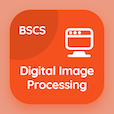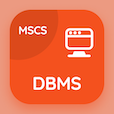Computer Science Courses Online

Digital Image Processing Practice Tests

Digital Image Processing Tests

The Book Wavelet Transforms in One Dimension Multiple Choice Questions (MCQ Quiz) PDF, Wavelet Transforms in One Dimension MCQs download to practice online digital image processing tests. Practice Wavelet and Multiresolution Processing Multiple Choice Questions and Answers (MCQs), Wavelet Transforms in One Dimension quiz answers PDF for top computer science schools in the world. The eBook Wavelet Transforms in One Dimension MCQ App Download: wavelet transforms in one dimension, multiresolution processing and wavelet, introduction to wavelet and multiresolution processing test prep for top online computer science programs.

The MCQ: FWT stands for PDF, "Wavelet Transforms in One Dimension MCQ" App Download (Free) with fast wavelet transformation, fast wavelet transform, fourier wavelet transform, and fourier wavelet transformation choices for top computer science schools in the world. Study wavelet transforms in one dimension quiz questions, download Google eBook (Free Sample) for online computer science engineering.

## Image Processing: Wavelet Transforms in One Dimension MCQs

MCQ: FWT stands for

A) Fast wavelet transformation
B) Fast wavelet transform
C) Fourier wavelet transform
D) Fourier wavelet transformation

MCQ: Wavelet series equation is the sum of

A) scaling coefficient
B) detail coefficient
C) span coefficient
D) Both A and B

MCQ: DWT stands for

A) Discrete wavelet transform
B) Discrete wavelet transformation
C) Digital wavelet transform
D) Digital wavelet transformation

MCQ: Scaling vectors are taken as

A) heights
B) sharpness
C) intensity
D) weights

MCQ: Heisenberg uncertainty principle is viewed as

A) tiles
B) blocks
C) squares
D) circles

### Practice Tests: Digital Image Processing Exam Prep

Download Digital Image Processing Quiz App, DBMS MCQ App, and DataBase Management System (MCS) MCQs App to install for Android & iOS devices. These Apps include complete analytics of real time attempts with interactive assessments. Download Play Store & App Store Apps & Enjoy 100% functionality with subscriptions!